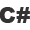Search for a lesson?

# Programming Tutorials - 63 Videos:### C# 315: Largest of a Set

Problem 14: How to find the largest number of any set of numbers.

9:18### C# 316: Palindrome

Problem 15 How to detect strings readable the same from both sides.

6:45### C# 317: Factorial

Problem 16: A typical loop exercise for multiplicative process in programming.

7:30### C# 318: Summation 1

Problem 17: How to solve mathematical summations in loops.

12:20### C# 319: Summation 2

Problem 18: How to solve mathematical summations in loops.

13:44### C# 320: Summation 3

Problem 19: How to solve mathematical summations in loops.

5:20### C# 321: Binary to Decimal

Problem 20: How to convert a binary number to decimal.

13:16### C# 322: Decimal to Binary

Problem 21: How to convert a decimal number to binary.

10:28

Page: 1 - 2 - 3 - 4 - 5 - 6 - 7 - 8